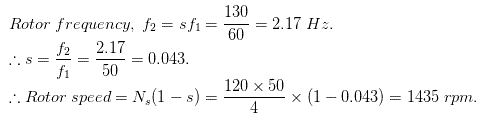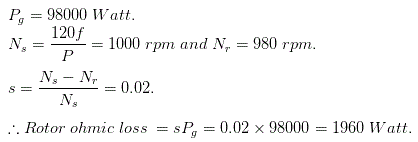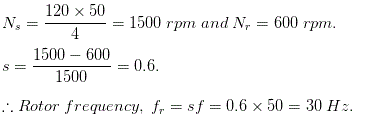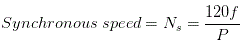# MCQs on Electrical Machines

##### Page 89 of 114. Go to page 1 2 3 4 5 6 7 8 9 10 11 12 13 14 15 16 17 18 19 20 21 22 23 24 25 26 27 28 29 30 31 32 33 34 35 36 37 38 39 40 41 42 43 44 45 46 47 48 49 50 51 52 53 54 55 56 57 58 59 60 61 62 63 64 65 66 67 68 69 70 71 72 73 74 75 76 77 78 79 80 81 82 83 84 85 86 87 88 89 90 91 92 93 94 95 96 97 98 99 100 101 102 103 104 105 106 107 108 109 110 111 112 113 114
01․ What does happen if tripled harmonics given to induction motor?
Will results in short circuit.
Fail to start.
Nothing will happen.
Will results in open circuit.

Sinusoidal wave form is an ideal form and is nearly impossible to realize such a wave form in practice. All the alternating wave forms deviate to a lesser or higher degree from this ideal sinusoidal shape. When a sinusoidal input is applied to electronic circuit the output is distorted wave form. This distorted wave is because of nonlinear characteristics of electronic circuit. Those distorted wave is called harmonics. The component having triple times of the fundamental are known as third harmonics. Generally all third harmonics are equal in all phases. These harmonics is applied to induction motor, it will fail to start because rotating magnetic field never develops. Hence it results in fail to start.

02․ Considering a galvanometer is connected to the rotor of a 3 - ÃŽÂ¦, 4 pole, 50 Hz IM and is making 130 oscillations in a minute, then calculate the rotor speed?
1435.
1440.
1450.
1500.

Since the galvanometer is connected to the rotor, one complete of galvanometer will correspond to 1 cycle of rotor frequency.03․ A 3 - ÃŽÂ¦, 6 pole, 50 Hz IM is running at a full load speed of 980 rpm. If it delivers an air gap power of 98000 Watt, calculate the rotor ohmic loss?
980 Watt.
1800 Watt.
1920 Watt.
1960 Watt.04․ Which of these types of motor is self starting?
Single phase induction motor.
Poly-phase induction motor.
Poly-phase synchronous machine.
None of these.

In a 3 - φ IM, 3 phase rotating flux is produced when energized from a 3 phase source. The 3 phase rotating flux cuts the stationary rotor windings and as a result, emf is produced. Due to this rotor induced emf, an current flows through the short-circuited rotor bars. This current interacts with the 3 - φ rotating flux and produces rotating torque, as a result the rotor starts rotating at a speed below synchronous speed. Hence, it is called self-starting as it starts without any auxiliary means.

05․ A 3 - ÃŽÂ¦, 4 pole IM is supplied from a 50 Hz source. Calculate rotor frequency when rotor runs 600 rpm?
30 Hz.
35 Hz.
20 Hz.
Can be determined.06․ What happens if fifth multiples of harmonics is given to induction motor?
It will rotates in reverse direction.
Fail to start.
It will rotates in normal operation.
Either short circuit or open circuit occurs.

The sinusoidal components having frequency five times of fundamental are known as fifth harmonics. All the fifth harmonics have a phase displacement of 120° and having a negative phase sequence of R, B, Y ( opposite rotation to the fundamental ). The fifth multiples of harmonics are 5th, 11th, 17th, 23rd etc. Hence induction motor will rotates in reverse direction because of reverse phase sequence of fifth harmonics.

07․ Rotating magnetic field is produced in an induction motor because of
stator winding.
rotor winding.
either stator winding or rotor winding.
none of the above.

Rotating magnetic field is developed in induction motor. Why? In order to develop rotating magnetic field the induction motor, it needs 120° phase shift of supply and winding in stator winding.

08․ The reduced air gap results in an increase
cost of operation.
breaking torque.
starting torque.
power factor.

As the air gap increased between stator and rotor it will increase magnetizing current and hence it results in low power factor. Hence a reduced air gap results in an increase power factor. Increase in magnetizing current is not desirable in any AC machine.

09․ Under operating conditions the rotor conductor should be _________________ in induction motor.
always closed.
always opened.
semi closed or semi opened.
none.

Current will flow only when after the formation of closed path. Under operating conditions rotor circuit of an induction motor should always be closed otherwise current can not flow hence no torque is produced.

10․ The synchronous speed of an induction motor will be increased by
decreasing of frequency.
increasing number of poles.
increasing of frequency.
speed can not be increased.Frequency is proportional to synchronous speed. Hence synchronous speed will increases as frequency increases.

<<<8788899091>>>